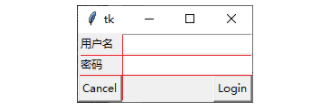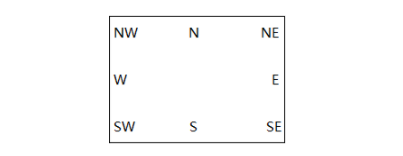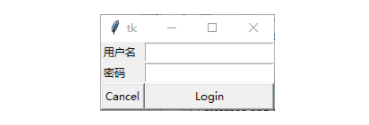• 写文章
• 提问

# python tkinter中的grid布局是什么？grid布局管理器的工作方式就是设想将父窗口空间划分为网格，并在网格中布置控件。```from tkinter import *
root = Tk()
Label(root, text="用户名").grid(row=0, sticky=W)
Label(root, text="密码").grid(row=1, sticky=W)
Entry(root).grid(row=0, column=1, sticky=E)
Entry(root).grid(row=1, column=1, sticky=E)
Button(root, text="Cancel").grid(row=2, column=0, sticky=E)
root.mainloop()```

row：指定控件所在行，缺省值为下一行。

column:指定控件所在列，缺省值为0

sticky:可选值包括NW，N，NE，W，E，SW，S，SE。这些值可以理解为地图上的方位，例如NE就是东北。`Button(root, text="Login").grid(row=2, column=1, sticky=E+W)`